# javascript基础的查缺补漏

### 对象转基本类型

let a = {
valueOf() {
return 0;
},
toString() {
return '1';
},
[Symbol.toPrimitive]() {
return 2;
}
}
1 + a // => 3
'1' + a // => '12'


'a'++'b'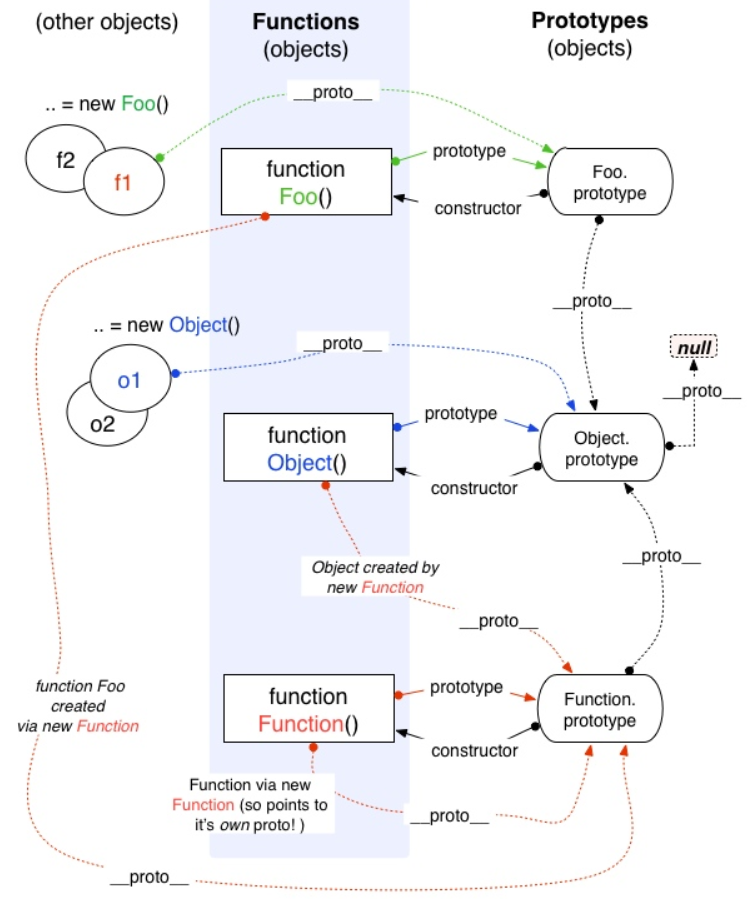function Foo() {
return this;
}
Foo.getName = function () {
console.log('1');
};
Foo.prototype.getName = function () {
console.log('2');
};

new Foo.getName();   // -> 1
new Foo().getName(); // -> 2

new (Foo.getName());
(new Foo()).getName();
//new Foo() 是返回return的内容而不是去看构造函数的属性


### []false, !![]true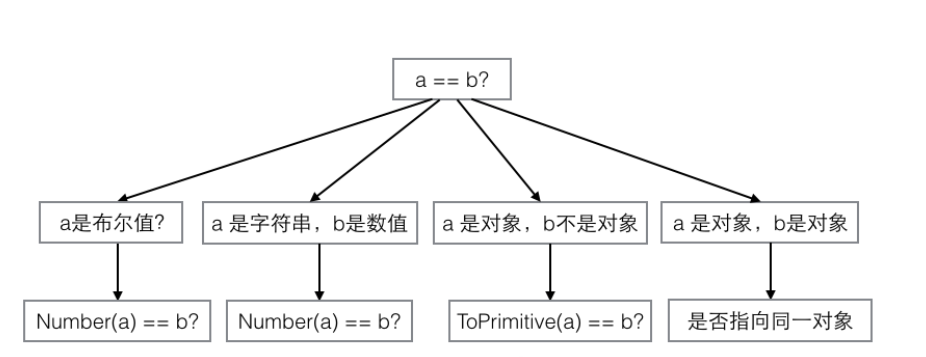js中隐式强制类型转换

NaN==NaN  false

Boolean() 判断 0、-0、null、false、NaN、undefined、或者空字符串（""），则生成的 Boolean 对象的值为 false,
![] 变成!Boolean([]) 也就是!true,也就是false


### [] + {} 和 {} + []一样吗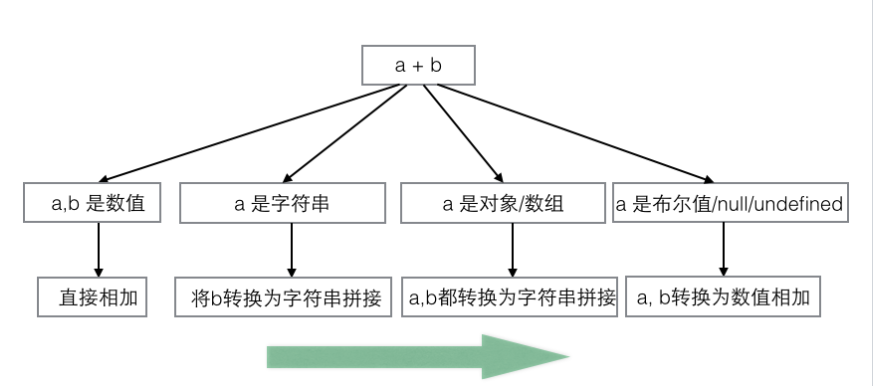• 如果两个操作数都是字符串,将两个字符串拼接起来
• 如果只有一个操作符石字符串,则另一个操作数转换为字符串(toString)

[]+{}

满足a有对象/数组,所有都转换为字符串拼接
""+"[object object]"="[object Object]"


1+{}

满足第三条 a是对象/数组
"1[object Object]"


{}+[]

"[object Object]"+toNumber([])
"[object Object]"+0


{}+{}

"[object Object][object Object]"


### 函数的name属性

通过构造函数方式创建的函数name都是 'anonymous'

function ff(){};
var f=ff.bind();
f.name 是bound ff


### 闭包

function F1(){
var a = 100
//返回一个函数(函数作为返回值)
return function(){
console.log(a)
}
}


function F1(){
var a = 100
return function(){
console.log(a)   //自由变量，父作用域寻找
}
}


• 全局变量
• 不销毁作用域
function fn(){
var a=1;
return function(){
a++
}
}


全局:关闭浏览器的时候销毁

var 是没有块级作用域的


是一个查找过程: 在一个私有作用域下遇到变量了,先看是不是自己私有的,如果不是往上一级找,没有继续找一只找到window下为之,没有就报错


看当前整个作用域对应我的地址是在哪一个作用域下定义的,那个作用域就是当前这个作用域的上一级


{}   if(){}    for(){}   while(){}


let 和const 定义的变量属于一个私有作用域,变量是私有变量

常见的变量


### javaScript 引擎

javaScript引擎是谷歌的v8引擎,这个引擎由两个部分组成

• 内存堆:这是内存分配发生的地方
• 调用栈:这是你的代码执行的地方

• 创建阶段
• 执行阶段

• this值的决定,就是this绑定
• 创建词法环境组件
• 创建变量组件

this的绑定

• 环境记录器
• 一个外部环境的引用

• 在全局环境中,环境记录器是对象环境记录器
• 在函数环境中,环境记录器是声明式环境记录器

### 值类型

// 值类型：Number、string、bollean、undefined
var a = 100
var b = a
a = 200
console.log(b) // 100 保存与复制的是值本身
typeof abc      //"undefined"
typeof null    //"object"

typeof区分不了引用类型(除了函数)

alert(person instanceof Object); // 变量 person 是 Object 吗？

alert(colors instanceof Array); // 变量 colors 是 Array 吗？

alert(pattern instanceof RegExp); // 变量 pattern 是 RegExp 吗？


### 引用传值

function addNum(num)
{
num+=10;
return num;
}
var num=10;
console.log(num);//10
console.log(result);//20

function mutate(obj) {
obj.a = true;
}

const obj = {a: false};
mutate(obj)
console.log(obj.a); // 输出 true

function setName(obj){
obj.name = '嘉明';
obj = new Object();
obj.name = '庞嘉明';
}
var person = new Object();
setName(person);
console.log(person.name); // '嘉明'，为啥不是'庞嘉明'呢？



### 当取值为百分比时，需要特别注意：百分比不是相对于父元素的高度的，而是相对于父元素的宽度的。height、top的百分比取值，总是相对于父元素的高度。

padding-topmargin-toppadding-bottommargin-bottom取值为百分比时，是相对于父元素的宽度

### fixed问题

一提到position:fixed，自然而然就会想到：相对于浏览器窗口进行定位。



if语句

### 为什么 ++[[]][+[]]+[+[]] = 10？

拆分
++[[]][+[]]
+
[+[]]

++[[]]
+


+([] + 1)
+


+([] + 1) === +("” + 1)，并且
+("” + 1) === +("1")，并且
+("1") === 1

1+
[]==''  =='0', =='1'



### 2==true为什么是false

'2'true '2' 隐式转化为2 2true 为false

### null >=0 ? true：false

null == 0  // false  null在设计上，在此处不尝试转型. 所以 结果为false.
null > 0  // false   null 尝试转型为number , 则为0 . 所以结果为 false,
null >= 0  // true
null<=0		//true

-null == 0  // true
+null == 0 // true
Number(null) == 0  // true


### 你不知道的JavaScript续集

var a = [ ];
a = 1;
a["foobar"] = 2;
a.length; // 1
a["foobar"]; // 2
a.foobar; // 2

var a = [ ];
a["13"] = 42;
a.length; // 14

var a = { '0': 1, '1': 2, '2': 3, length: 3 };
function foo() {
var arr = Array.prototype.slice.call(arguments);
arr.push("bam");
console.log(arr);
}
foo("bar", "baz"); // ["bar","baz","bam"]



JavaScript中字符串是不可变的，而数组是可变的。

var a=2/'foo';
a==NaN //false

ES6开始我们使用Number.isNaN()
var a = 2 / "foo";
var b = "foo";
Number.isNaN( a ); // true
Number.isNaN( b ); // false——好！

var a = 1 / 0; // Infinity
var b = -1 / 0; // -Infinity

var a = 0 / -3; // -0
var b = 0 * -3; // -0

+"-0"; // -0
Number( "-0" ); // -0
JSON.parse( "-0" ); // -0



var a = [1,2,3];
var b = a;
a; // [1,2,3]
b; // [1,2,3]
// 然后
b = [4,5,6];
a; // [1,2,3]
b; // [4,5,6]

function foo(x) {
x.push(4);
x; // [1,2,3,4]
// 然后
x = [4, 5, 6];
x.push(7);
x; // [4,5,6,7]
}
var a = [1, 2, 3];
foo(a);
a; // 是[1,2,3,4]，不是[4,5,6,7]

foo(a.slice())

function foo(wrapper) {
wrapper.a = 42;
}
var obj = {
a: 2
};
// var obj=new Object();  obj.a=2;
foo(obj);
obj.a; // 42

function foo(x) {
x = x + 1;
x; // 3
}
var a = 2;
var b = new Number(a); // Object(a)也一样
foo(b);
console.log(b); // 是2，不是3



var a=Boolean(false);
var b=new Boolean(false);
if (!b) {
console.log( "Oops" ); // 执行不到这里
}

var a = "abc";
var b = new String( a );
var c = Object( a );
typeof a; // "string"
typeof b; // "object"
typeof c; // "object"
b instanceof String; // true
c instanceof String; // true


可以使用ValueOf()函数
var a = new String( "abc" );
var b = new Number( 42 );
var c = new Boolean( true );
a.valueOf(); // "abc"
b.valueOf(); // 42
c.valueOf(); // true

var a = new String( "abc" );
var b = a + ""; // b的值为"abc"
typeof a; // "object"
typeof b; // "string"


关于数组（array）、对象（object）、函数（function）和正则表达式，我们通常喜欢以常量的形式来创建它们。实际上，使用常量和使用构造函数的效果是一样的（创建的值都是通过封装对象来包装）

var a = new Array( 1, 2, 3 );
a; // [1, 2, 3]
var b = [1, 2, 3];
b; // [1, 2, 3]

Array构造函数只带一个数字参数的时候，该参数会被作为数组的预设长度（length），而非只充当数组中的一个元素



var a = 42;
var b = a++;
a; // 43
b; // 42 这是a++的副作用

========================
function foo() {
a = a + 1;
}
var a = 1;
foo(); // 结果值：undefined。副作用：a的值被改变


### 你懂 JavaScript 嗎？

toJSON

var obj = {
key: 'foo',
toJSON: function () {
return 'bar';
}
};
var ret = JSON.stringify(obj);
console.log(ret);



Number

undefined   ---> NaN
null		---> 0
boolean 的true为1  false即是0
string  -->数字或NaN
object		若定义valueOf优先用,其次toString

Number(undefined) // NaN
Number(null) // 0
Number(true) // 1
Number(false) // 0
Number('12345') // 12345
Number('Hello World') // NaN
Number({ name: 'Jack' }}) // NaN

const a = {
name: 'Apple',
valueOf: function() {
return '999'
}
}

Number(a) // 999

const a = new String('');
const b = new Number(0);
const c = new Boolean(false);

!!a // true
!!b // true
!!c // true


parseInt

参数:第一个参数是string 第二个参数是介于2和36之间的整数,通常默认为10,也就是我们通常使用的十进制转换,如果是5就是5进制,超出这个范围，则返回NaN。如果第二个参数是0、undefined和null，则直接忽略
* 将字符串转为整数
* 如果字符串头部有空格,空格会被自动去除
* 如果参数不是字符串,先转为字符串再转换
parseInt('12px')  如果遇到不能转为数字的字符,就不再进行下去,返回转好的部分



const a = true;
const b = 123;

a === b // false
a == b // false
true强制转换为1

const a = '1,2,3';
const b = [1,2,3];
a === b // false
a == b // true

Object(null) 和Object(undefined) 等同于Object()也就是{}
var a = null;
var b = Object(a); // 等同於 Object()
a == b; // false

var c = undefined;
var d = Object(c); // 等同於 Object()
c == d; // false

var e = NaN;
var f = Object(e); // 等同於 new Number(e)
e == f;//false

Number.prototype.valueOf = function() {
return 3;
};

new Number(2) == 3; // true


• a <= b其实是!(b > a)，因此!false得到true。
• a >= b其实是b <= a也就是!(a > b)等同于!false得到true
const a = { b: 12 };
const b = { b: 13 };

a < b // false，'[object Object]' < '[object Object]'
a > b // false，其實是比較 b < a，即 '[object Object]' < '[object Object]'
a == b // false，其實是比較兩物件的 reference

a >= b // true
a <= b // true

[]==[]  false 因为两个的地址不是一样的

'ab' < 'cd' // true   以字典的字母顺序形式进行比较

'Hello World' > 1 // false，字串 'Hello World' 无法转化为数字,变成了NaN
NaN 不大于、不小于、不等于任何值，当然也不等于自己


### 图解构造器Function和Object的关系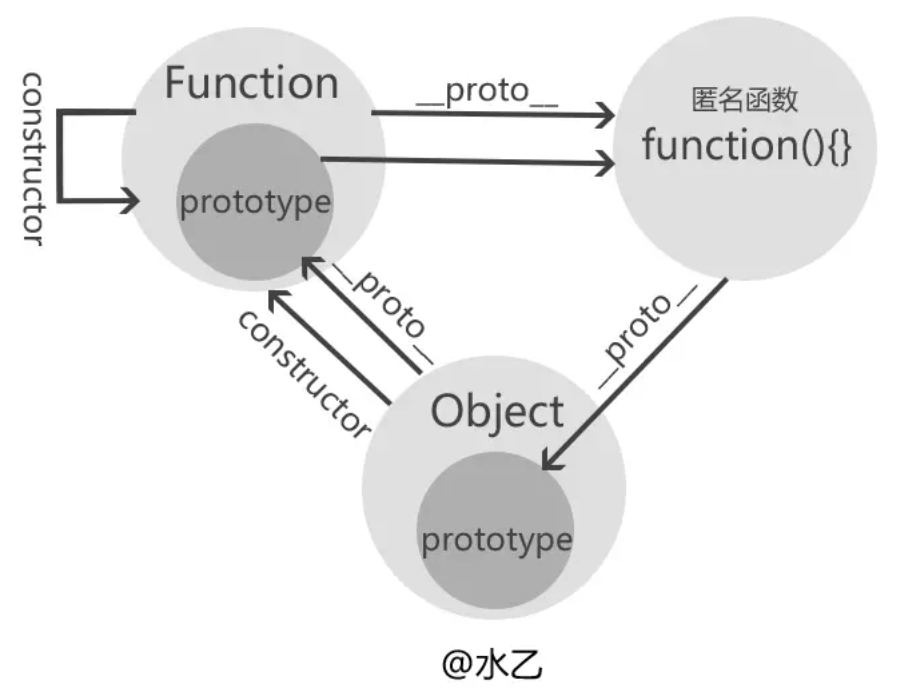//①构造器Function的构造器是它自身
Function.constructor=== Function;//true

//②构造器Object的构造器是Function（由此可知所有构造器的constructor都指向Function）
Object.constructor === Function;//true

//③构造器Function的__proto__是一个特殊的匿名函数function() {}
console.log(Function.__proto__);//function() {}

//④这个特殊的匿名函数的__proto__指向Object的prototype原型。
Function.__proto__.__proto__ === Object.prototype//true

//⑤Object的__proto__指向Function的prototype，也就是上面③中所述的特殊匿名函数
Object.__proto__ === Function.prototype;//true
Function.prototype === Function.__proto__;//true


Function instanceof Object 和 Object instanceof Function 运算的结果当然都是true啦

Function 和prototype指向一个特殊匿名函数,而这个特殊匿名函数的 __proto__ 指向 Object.prototype

### Array.of

Array.of方法用于将一组值，转换为数组。
Array.from()
Array.from方法用于将两类对象转为真正的数组：类似数组的对象和可遍历（iterator）的对象（包括Map和Set）


### 创建包含N个空对象的数组

Array(3).fill().map(()=>({}))
Array.apply(null,{length:3}).map(()=>({}))


### 函数表达式

函数表达式，则必须等到解析器执行到它所在的代码行，才会真正被解析。
console.log(sum(10 , 10)); //TypeError: sum is not a function
var sum = function(num1 , num2){
return num1 + num2;
}


### Javascript中Y组合子

递归就是函数不断调用自身

let factorial=n=>n?factorial(n-1)*n:1;

const factorial = n => n === 1 ? 1 : n * factorial(n - 1)

const factorial = (n, total) => n === 1 ? total : factorial(n - 1, n * total)

const fact = (n, total = 1) => n === 1 ? total : fact(n - 1, n * total)

Lambda函数(匿名函数)
// ES5
var f = function (x) {
return x;
};

// ES6
const f = x => x
lambda表达式写出递归(匿名函数递归)

const factorial= (f,n) => n===1 ? 1 : n*f(f,n-1);
factorial(factorial,6)
//这个也太难看了,解决方案柯里化
// 这块不怎么好懂我就忽略了

Lambda演算
Lambda演算中所有函数式匿名的,它们没有名称,只接受一个输出变量,即独参函数

const invokeWithSelf = f => f(f)

const fact = (n, total = 1) => n === 1 ? total : fact(n - 1, n * total)

const fact = f => (total = 1) => n => n === 1 ? total : f(f)(n * total)(n - 1)
const factorial = fact(fact)()

factorial(6) // => 720

const fact = f => (total = 1) => n => n === 1 ? total : f(n * total)(n - 1)

const Y = f => (x => f(v => x(x)(v)))
(x => f(v => x(x)(v))) // 瞧，这不就是黑魔法Y组合子嘛

const factorial = Y(fact)()

factorial(6) // => 720


### 尾调用优化

尾调用时指在函数return的时候调用一个新的函数,由于尾调用的实现需要存储到内存中,在一个循环体中，如果存在函数的尾调用，你的内存可能爆满或溢出。

const factorial = (n, total) => n === 1 ? total : factorial(n - 1, n * total)


### let

let和const都能够声明块级作用域,let的特点是不会变量提升，而是被锁在当前块中。
function test() {
if(true) {
console.log(a)//TDZ，俗称临时死区，用来描述变量不提升的现象
let a = 1
}
}
test()  // a is not defined

function test() {
if(true) {
let a = 1
}
console.log(a)
}
test() // a is not defined

临时死区的意思是在当前作用域的块内，在声明变量前的区域叫做临时死区。


### Object.is()

用来解决JavaScript中特殊类型 == 或者 === 异常的情况。
Object.is()来处理2个值的比较。
console.log(Object.is(NaN, NaN)) // true
console.log(Object.is(+0, -0)) // false
console.log(Object.is(5, "5")) //false


### 解构赋值

function test(value) {
console.log(value);
}
test({a=1,b=2}={a:2,b:3});


### yield使用限制

yield只可以在生成器函数内部使用，如果在非生成器函数内部使用，则会报错。
function *createIterator(items) {
//你应该在这里使用yield
items.map((value, key) => {
yield value //语法错误，在map的回调函数里面使用了yield
})
}
const a = createIterator([1, 2, 3]);
console.log(a.next()); //无输出

const obj = {
a: 1,
*createIterator() {
yield this.a
}
}
const a = obj.createIterator();
console.log(a.next());  //{value: 1, done: false}


### 函数的caller

caller : 当前这个函数在哪个函数调用的
function fn(){
console.log(fn.caller);
}
function ff() {
fn();
}
ff();//[Function: ff]
arguments.callee  就是当前函数本身
function fn(){
console.log(argument.callee)
}
fn.prototype.constructor===fn;//true  ,也代表的是函数本身


### 捕获和冒泡

xxx.onclick=function(){} //DOM0事件绑定,给元素的事件行为绑定方法,这些方法在事件传播的冒泡阶段(或者目标阶段)执行的
//第三个参数false也是控制绑定的方法在事件传播的冒泡阶段执行,但是在捕获阶段执行没有实际意义,默认是false,可以不写


### DOM0和DOM2的运行机制

DOM0事件绑定的原理:就是给元素的某一个事件私有属性赋值(浏览器会建立监听机制,当我们出发元素的某个行为,浏览器会自己把属性中赋的值去执行)

DOM0事件绑定:只允许给当前元素的某个事件行为绑定一个方法,多次绑定后面的内容会替换前面绑定的,以最后一次绑定的方法为主

DOM0事件绑定和DOM2事件绑定的区别

• DOM0采用的是私有属性赋值,所有只能绑定一个方法
• DOM2采用的是事件池机制,所以能绑定多次方法

    let list = document.querySelector('#list');
console.log(ev.target.innerHTML);
})
console.log(2);
})

box.onclick=function(){}
box.onclick=null// DOM0的移出(不需要考虑绑定的是谁)

//DOM2移出的时候
function f3() {
console.log(2);
}
list.removeEventListener('click',f3);
//DOM2移出的时候,必要清除移出的是哪个方法技巧(不要绑定匿名函数,都绑定实名函数)


DOM0和DOM2是可以同时使用,因为是浏览器的两个运行机制,执行顺序和编写顺序有关

### mouseenter和mouseover的区别

1. over属于滑过事件,从父元素进入子元素,属性离开父亲,会触发父元素的out,触发子元素的over
enter属于进入,从父元素进入子元素,并不算离开父元素,不会触发父元素的leave,触发子元素的enter
2. enter和leave阻止了事件的冒泡传播,而over和out还存在冒泡传播的



### 事件委托(事件代理)

<ul id="list">
<li>item 1</li>
<li>item 2</li>
<li>item 3</li>
<li>item n</li>
</ul>
<script>
let list = document.querySelector('#list');
list.onclick=function (ev) {
let target=ev.target||window.event.target;
console.log(target.innerHTML);
}
</script>


### JQ的事件绑定

on/off : 基于DOM2事件绑定实现事件的绑定和移除

one:只绑定一次,第一次执行完成后,会把绑定的方法移出(基于on/off完成)

click/ mouseenter/... jq提供的快捷绑定方法,但是这些方法都是基于on/off完成的

delegate 事件委托方法(在1.7以前用的是live方法)

$(document).on('click',fn)$(document).off('click',fn)
$(document).one('click',fn)$(document).click(fn)


### 自执行匿名函数

(function(){ /* code */ }());


### forEach和map的区别

• forEach和map方法里每次执行匿名函数都支持3个参数，参数分别是item（当前每一项）、index（索引值）、arr（原数组）

map

forEach

### Promise A+规范

class Promise {
constructor(excutorCallBack) {
this.status = 'pending';
this.value = undefined;
this.fulfilledAry = [];
this.rejectedAry = [];
let resolveFn = result => {
let timer=setTimeout(()=>{
clearTimeout(timer);
if (this.status !== 'pending') return;
this.status = 'fulfilled';
this.value = result;
this.fulfilledAry.forEach(item=>item(this.value))
})
};
let rejectFn = reason => {
let timer=setTimeout(()=>{
if (this.status !== 'pending') return;
this.status = 'rejected';
this.value = reason;
this.rejectedAry.forEach(item => item(this.value));
})
};
try{
excutorCallBack(resolveFn, rejectFn());
}catch(err){
// 有异常信息按照rejected状态处理
rejectFn(err);
}
excutorCallBack(resolveFn, rejectFn);
}

then(fulfilledCallBack, rejectedCallBack) {
//处理不传递的状况
typeof fulfilledCallBack!=='function'?fulfilledCallBack=result=>result:null;
typeof rejectedCallBack!=='function'?rejectedCallBack=reason=>{
throw new Error(reson.message);
}:null;
//返回一个新的promise实例
return new Promise((resolve,reject)=>{
this.fulfilledAry.push(()=>{
try{
let x=fulfilledCallBack(this.value);
x instanceof Promise?x.then(resolve,reject):resolve(x);
// if(x instanceof Promise){
//     x.then(resolve, reject);
//     return;
// }
// resolve(x);
}catch(err){
reject(err)
}
});
this.rejectedAry.push(()=>{
try{
let x=rejectedCallBack(this.value);
x instanceof Promise?x.then(resolve,reject):resolve(x);
// resolve(x);
}catch(err){
reject(err)
}
});
});
// this.fulfilledAry.push(fulfilledCallBack);
// this.rejectedAry.push(rejectedCallBack);
}

catch(rejectedCallBack) {
return this.then(null,rejectedCallBack)
}

static all(promiseAry=[]){
return new Promise((resolve, reject)=>{
//index:记录成功的数量 result记录成功的结果
let index=0,
result=[];
for (let i = 0; i <promiseAry.length; i++) {
//promiseAry[i] 每一个需要处理的promise实例
promiseAry[i].then(val=>{
index++;
result[i]=val;
//索引需要和promiseAry对应,保证结果的顺序和数组的顺序一致
if (index === promiseAry.length) {
resolve(result);
}
}, reject);
}
});
}
}
module.exports = Promise;


### call,apply,bind串联起来理解

cat.call(dog, a, b) = cat.apply(dog, [a, b]) = (cat.bind(dog, a, b))() = dog.cat(a, b)


### 本地存储和服务器存储

• 页面之间的信息通信
• 性能优化

session是服务器存储

• 不兼容IE8及以下
• 也有存储的大小限制,一个源下最多只能存储5MB内容
• 本地永久存储,只要你不手动删除,永久存储在本地(但是我们可以基于API removeItem/clear手动清除)
• 杀毒软件或者浏览器的垃圾清除暂时不会清除localStorage(新版本谷歌会清除localStorage)
• 在隐私或者无痕浏览下,是记录localStorage
• localStorage和服务器没有半毛钱关系

• 兼容所有的浏览器
• 有存储的大小限制,一般一个源只能存储4kb内容
localStorage.gsetItem([key],[value])//[value]必须是字符串格式(即使写的不是字符串,也会默认转换为字符串)
localStorage.getItem([key]) //通过属性名获取存储的信息
localStorage.removeItem([key])//删除指定的存储信息
localStorage.clear()//清除当前域下存储的所有信息
localStorage.key(0)//基于索引获取指定的key名


### 数组的方法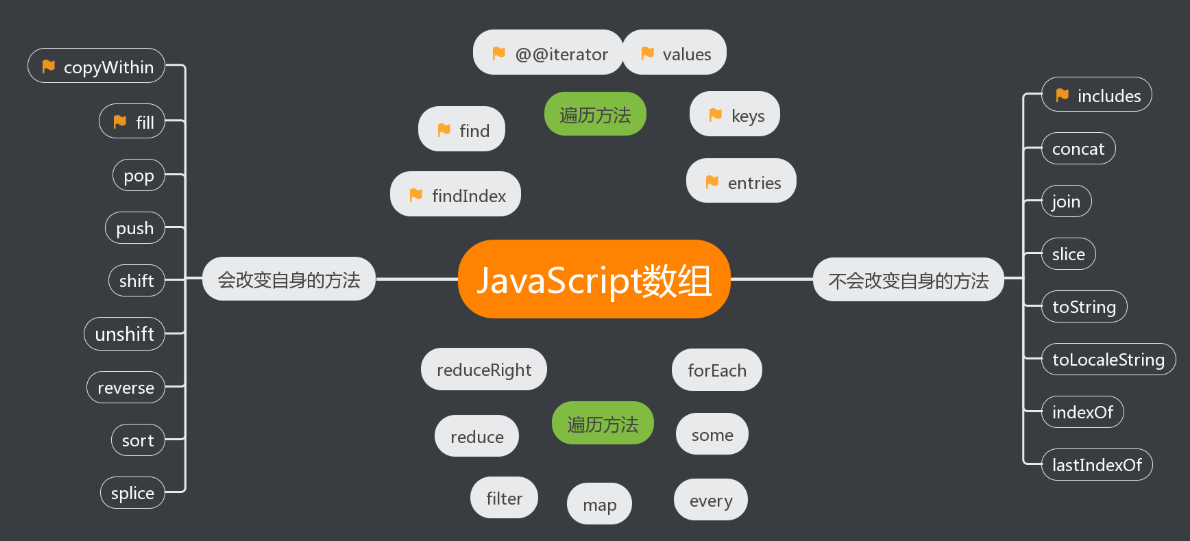### flex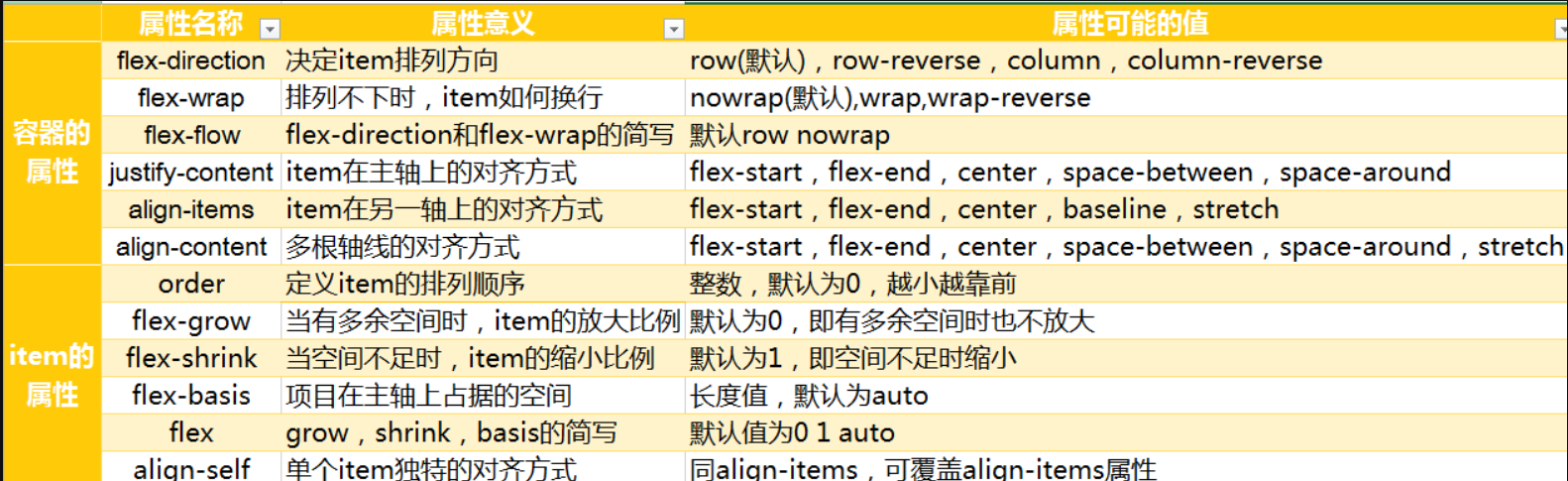需要一个容器 display:flex
flex-direction (元素排列方向)
row, row-reverse, column, column-reverse
flex-wrap (换行)
nowrap, wrap, wrap-reverse
flex-flow (以上两者的简写)
flex-direction || flex-wrap
justify-content (水平对齐方式)
flex-start, flex-end, center, space-between, space-around
align-items (垂直对齐方式)
stretch, flex-start, flex-end, center, baseline
align-content (多行垂直对齐方式)
stretch, flex-start, flex-end, center, space-between, space-around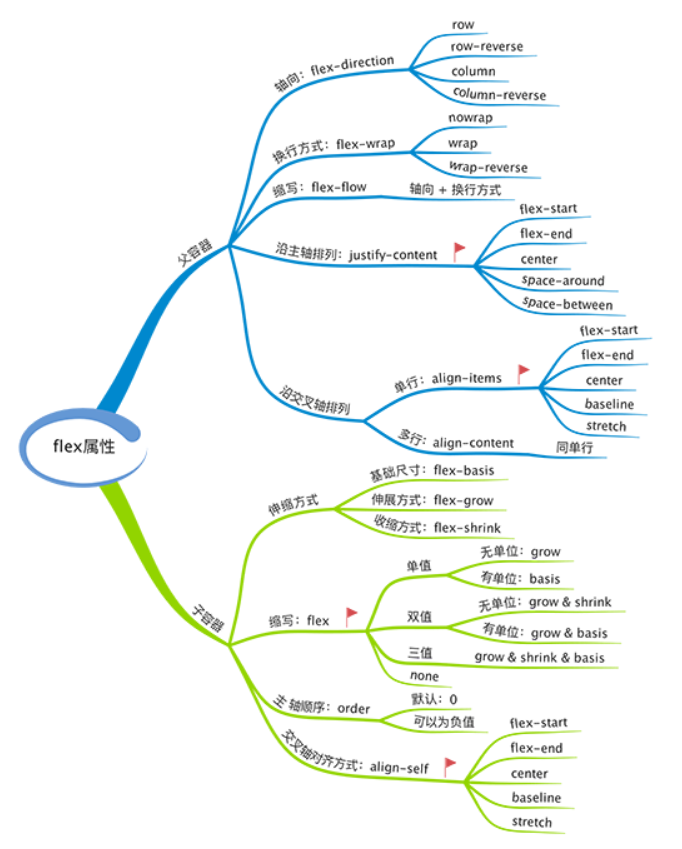### 性能优化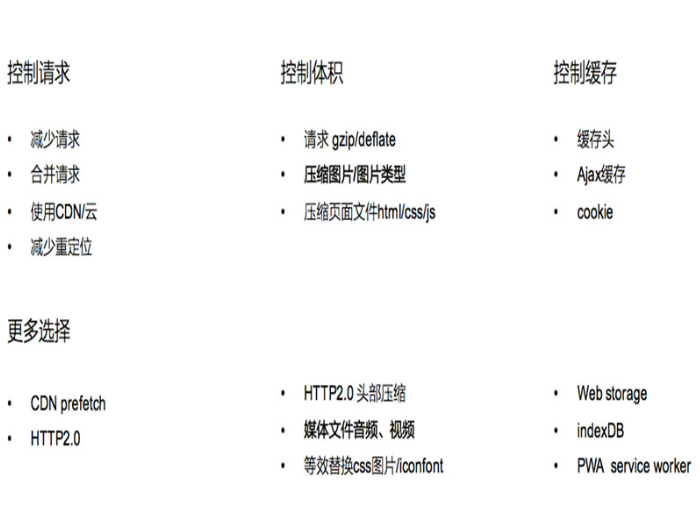DNS:负责将域名URL转化为服务器主机IP
DNS查找流程：首先查看浏览器缓存是否存在，不存在则访问本机DNS缓存，再不存在则访问本地DNS服务器。所以DNS也是开销，通常浏览器查找一个给定URL的IP地址要花费20-120ms，在DNS查找完成前，浏览器不能从host那里下载任何东西。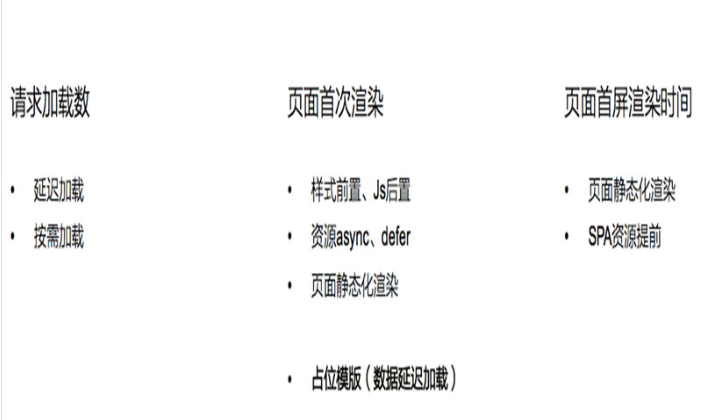defer

<script defer src='1.js'></script>
<script>
})
</script>


async

async的设置，会使得script脚本异步的加载并在允许的情况下执行 async的执行，

defer 如果你的脚本代码依赖于页面中的DOM元素(文档是否加载解析完毕),或者被其他脚本文件依赖

• 评论框 代码语法高亮• 就是浏览器直接输出渲染好数据的html页面(简称直出)
• 直出就是需要node.js的支持,服务器上的浏览器渲染好的东西,直接输出给客户端的浏览器
• 简单来说,就是直接把配件选好,让店家帮忙组装器,一次性发过来,就是直出这个道理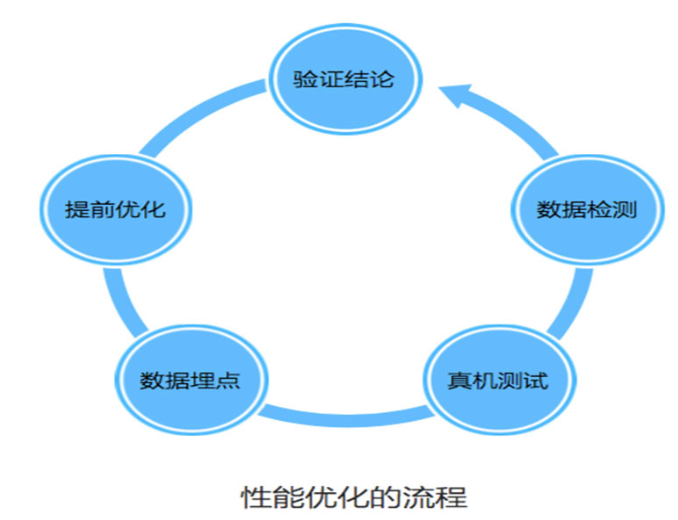### arguments对象是类数组

apply()方法接受两个参数:一个是运行函数的作用域,另一个是参数数组,这个参数数组可以是Array实例,也可以是arguments对象(类数组对象)

function sum(num1 , num2){
return num1 + num2;
}
function callSum1(num1,num2){
return sum.apply(this,arguments); // 传入arguments类数组对象
}
function callSum2(num1,num2){
return sum.apply(this,[num1 , num2]); // 传入数组
}
console.log(callSum1(10 , 10)); // 20
console.log(callSum2(10 , 10)); // 20


### Object.create()和new object()和{}的区别

Object.create()

• Object.create(null) 创建的对象是一个空对象，在该对象上没有继承 Object.prototype 原型链上的属性或者方法

• Object.create()方法接受两个参数:Object.create(obj,propertiesObject) ;

obj:一个对象，应该是新创建的对象的原型。

propertiesObject：可选。该参数对象是一组属性与值，该对象的属性名称将是新创建的对象的属性名称，值是属性描述符（这些属性描述符的结构与Object.defineProperties()的第二个参数一样）。注意：该参数对象不能是 undefined，另外只有该对象中自身拥有的可枚举的属性才有效，也就是说该对象的原型链上属性是无效的。

var o = Object.create(Object.prototype, {
// foo会成为所创建对象的数据属性
foo: {
writable:true,
configurable:true,
value: "hello"
},


### 从输入 url 到展示的过程

• DNS解析

• TCP三次握手

• 发送请求,分析url,设置请求报文(头,主体)

• 服务器返回请求的文件(html)

• 浏览器渲染

• html parse==>DOM Tree

标记化算法,进行元素状态的标记

dom树构建

• css parser==>Styel tree

解析css代码,生成样式树

• attachment==>Render Tree

结合dom树与style树,生成渲染树

• layout:布局

• GPU painting:像素绘制页面

### 内存泄露

• 意外地全局变量,无法被回收
• 定时器:未被正确关闭,导致所引用的外部变量无法被释放
• 事件监听:没有正确销毁
• 闭包:会导致父级中的变量无法被释放
• dom引用: dom元素被删除时,内存中的引用未被正确清空

• 新生成了一个对象
• 链接到原型
• 绑定this
• 返回新对象

### JQ的attr和prop的区别

• attr是通过setAttributegetAttribute来设置的,使用的是DOM属性节点
• prop是通过document.getElementById(el)[name]=vlaue来设置的,是转化为js对象的属性
• 通过设置checked，selected，readonly，disabled等的时候使用prop效果更好，减少了访问dom属性节点的频率。
• 一般如果是标签自身自带的属性，我们用prop方法来获取；如果是自定义的属性，我们用attr方法来获取。

### DOM节点的attr和property有何区别

• property只是一个JS对象的属性的修改
• Attribute是对html标签属性的修改

### 获取当前时间

new Date().toISOString().slice(0,10)


### toLocaleString()

var number = 1337;
var date = new Date();
var myArr = [number, date, "foo"];
var str = myArr.toLocaleString();
console.log(str);
// 输出 "1,337,2019/2/15 下午8:32:24,foo"

let a=3500
a.toLocaleString() //3,500


### 写React/Vue项目时为什么要在组件中写key,其作用是什么

key的作用是为了在diff算法执行时更快的找到对应的节点,提高diff速度

### 构造函数的返回值

• 没有返回值则按照其他语言一样返回实例化对象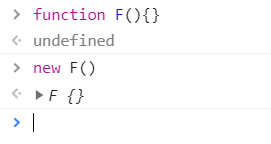• 若有返回值则检查其返回值是否为引用类型。如果是非引用类型，如基本类型（string,number,boolean,null,undefined）则与无返回值相同，实际返回其实例化对象。

• 若返回值是引用类型，则实际返回值为这个引用类型。

posted @ 2019-02-19 13:47  猫神甜辣酱  阅读(1129)  评论(1编辑  收藏